# (Solved) : Rsa Encryption Lab Please Someone Help Know Lab Said Work Partners M Dead Late Pick Phrase Q42702106 . . .

RSA ENCRYPTION LAB!!!

PLEASE, CAN SOMEONE HELP ME WITH THIS? I KNOW THIS LAB SAID TOWORK WITH PARTNERS, BUT I’M SO DEAD LATE. JUST PICK YOUR OWN PHRASEAND HELP ME PLEASE!!! THANK YOU!!

THE rsa.xls IS BELOW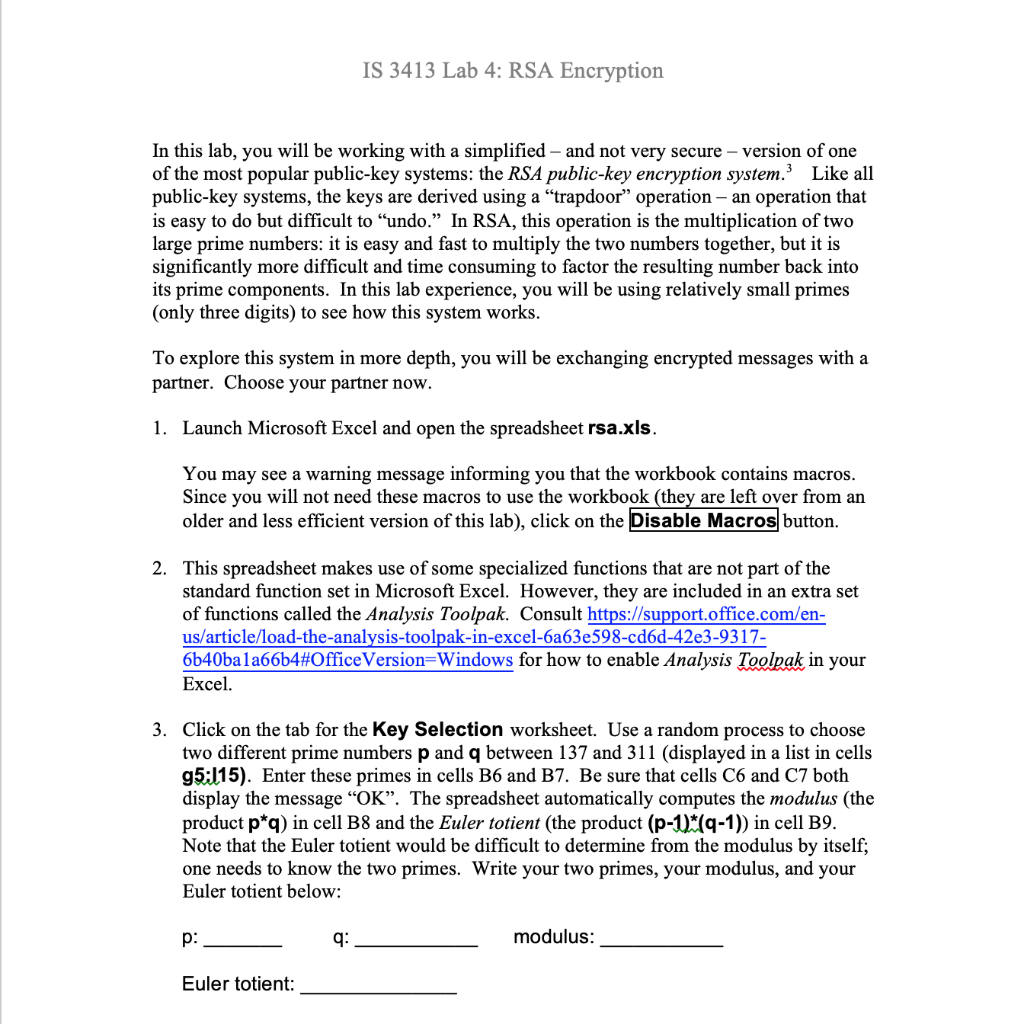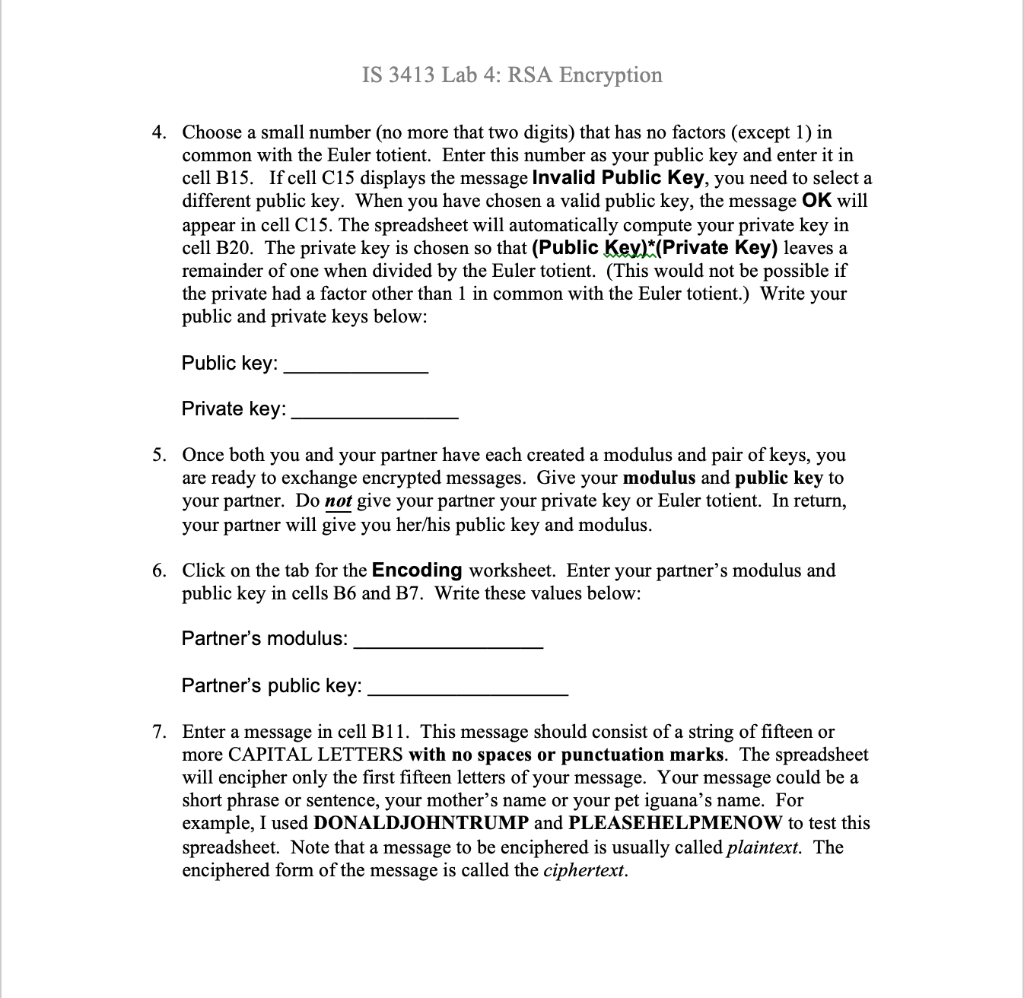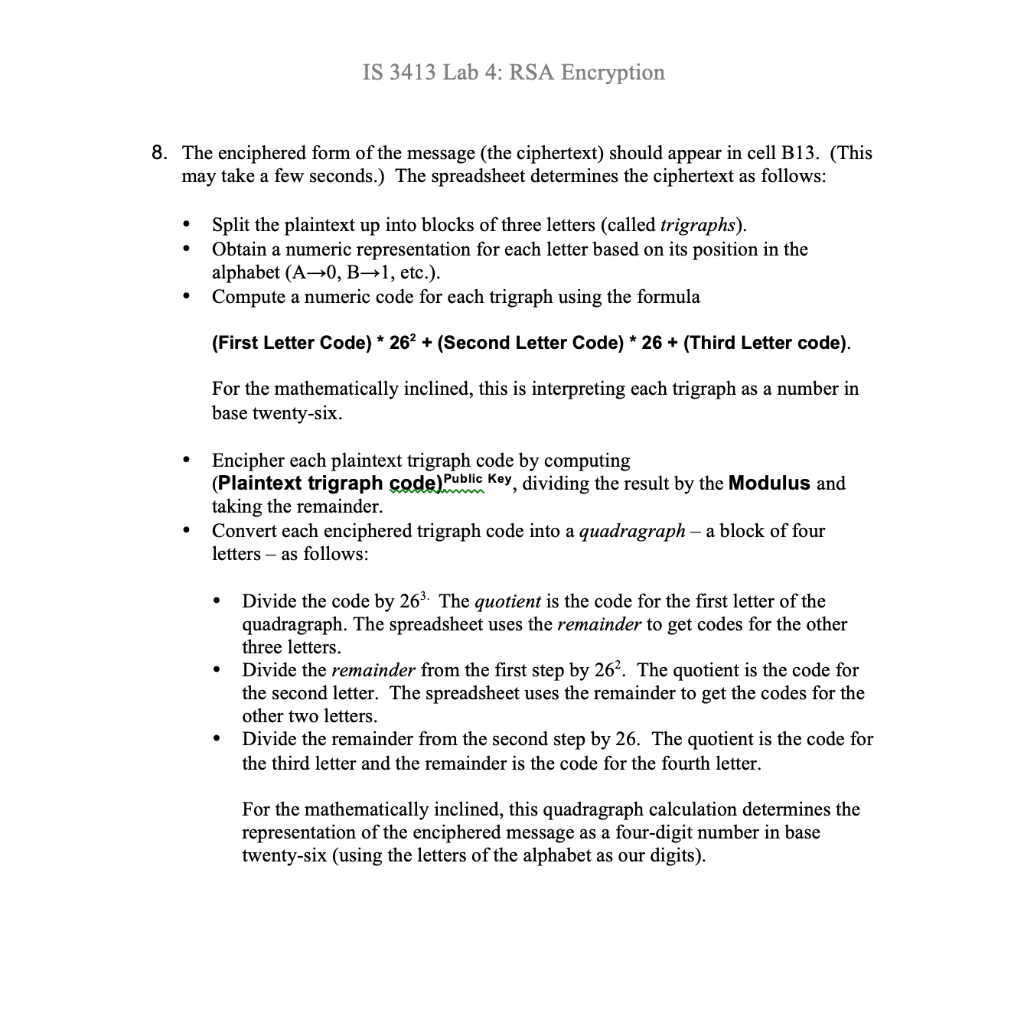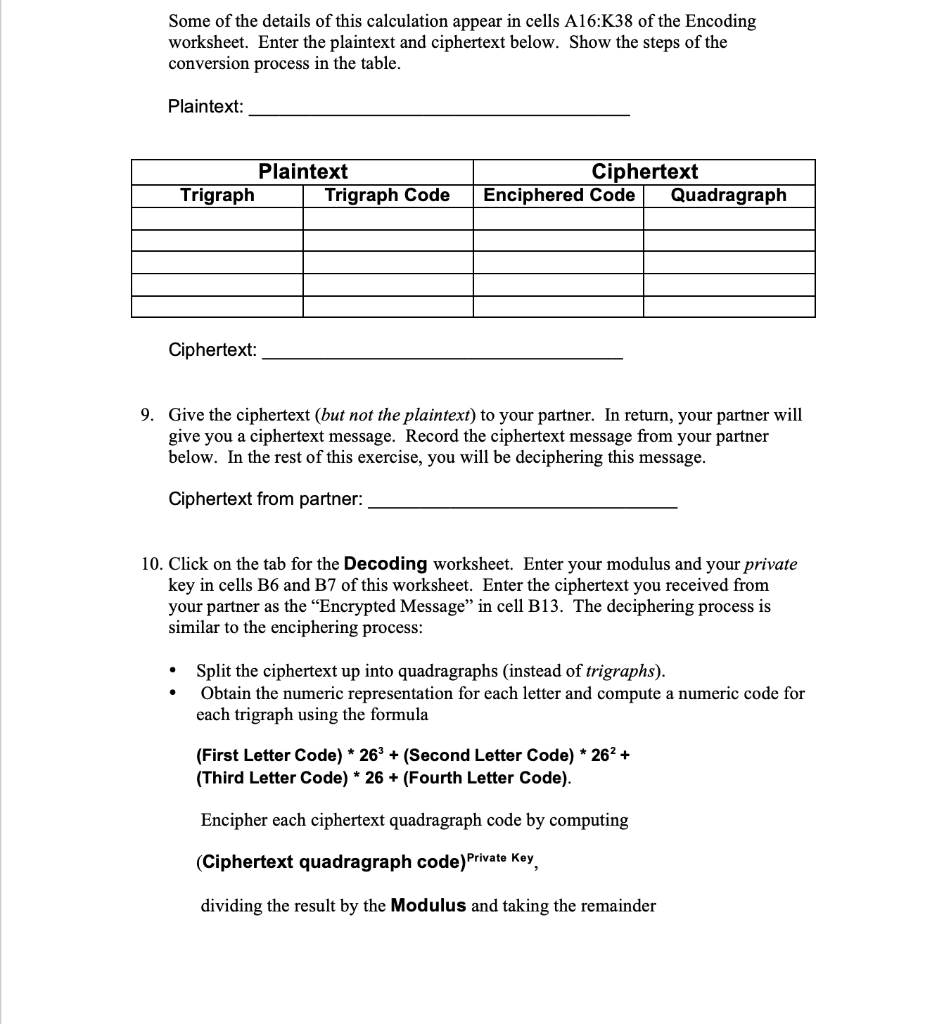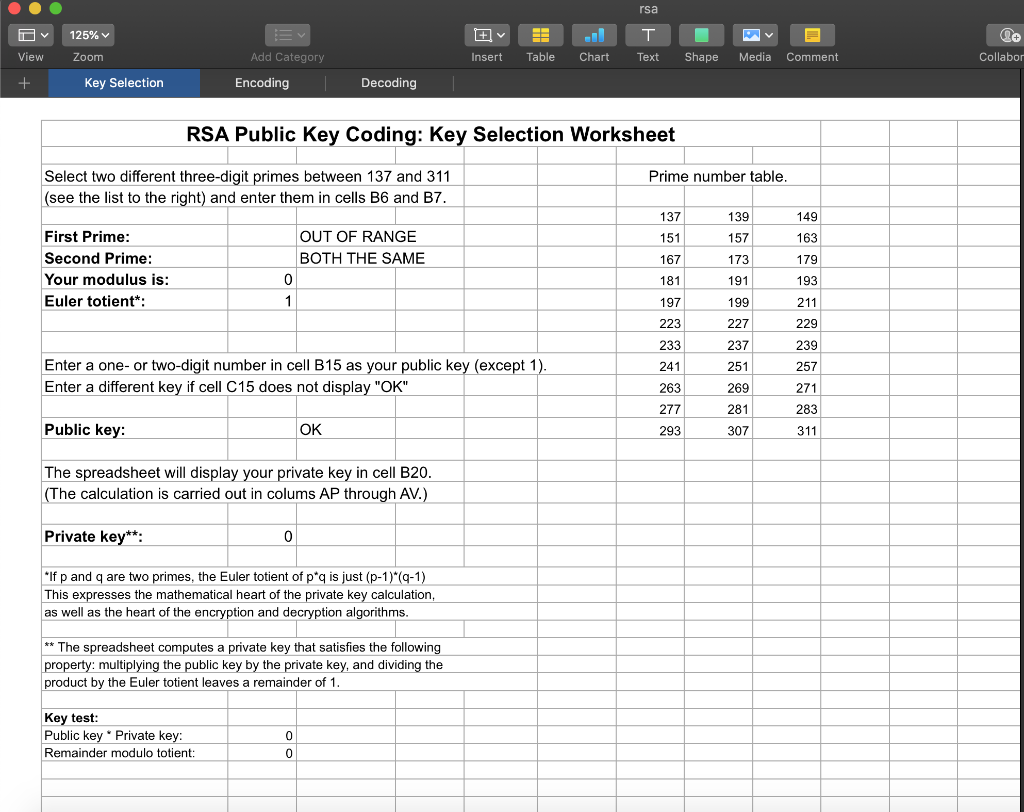IS 3413 Lab 4: RSA Encryption 8. The enciphered form of the message (the ciphertext) should appear in cell B13. (This may take a few seconds.) The spreadsheet determines the ciphertext as follows: • Split the plaintext up into blocks of three letters (called trigraphs). Obtain a numeric representation for each letter based on its position in the alphabet (A-0, B 1, etc.). Compute a numeric code for each trigraph using the formula (First Letter Code) * 262 + (Second Letter Code) * 26+ (Third Letter code). For the mathematically inclined, this is interpreting each trigraph as a number in base twenty-six. Encipher each plaintext trigraph code by computing (Plaintext trigraph code) Public Key, dividing the result by the Modulus and taking the remainder. Convert each enciphered trigraph code into a quadragraph – a block of four letters – as follows: Divide the code by 263. The quotient is the code for the first letter of the quadragraph. The spreadsheet uses the remainder to get codes for the other three letters. Divide the remainder from the first step by 26″. The quotient is the code for the second letter. The spreadsheet uses the remainder to get the codes for the other two letters. Divide the remainder from the second step by 26. The quotient is the code for the third letter and the remainder is the code for the fourth letter. For the mathematically inclined, this quadragraph calculation determines the representation of the enciphered message as a four-digit number in base twenty-six (using the letters of the alphabet as our digits).
O View + 125% Zoom Key Selection rsa Ev. Tv Insert Table Chart Text Shape Media Comment Add Category Collabor Encoding Decoding RSA Public Key Coding: Key Selection Worksheet Prime number table. Select two different three-digit primes between 137 and 311 (see the list to the right) and enter them in cells B6 and B7. 149 163 OUT OF RANGE BOTH THE SAME First Prime: Second Prime: Your modulus is: Euler totient*: 193 137 151 167 181 197 223 233 241 263 277 139 157 173 191 199 227 237 251 269 281 239 257 Enter a one-or two-digit number in cell B15 as your public key (except 1). Enter a different key if cell C15 does not display “OK” 283 Public key: OK 293 307 311 The spreadsheet will display your private key in cell B20. (The calculation is carried out in colums AP through AV.) Private key**: *If p and q are two primes, the Euler totient of p*q is just (p-1)*(4-1) This expresses the mathematical heart of the private key calculation, as well as the heart of the encryption and decryption algorithms. ** The spreadsheet computes a private key that satisfies the following property: multiplying the public key by the private key, and dividing the product by the Euler totient leaves a remainder of 1. Key test: Public key * Private key: Remainder modulo totient:

Answer to RSA ENCRYPTION LAB!!! PLEASE, CAN SOMEONE HELP ME WITH THIS? I KNOW THIS LAB SAID TO WORK WITH PARTNERS, BUT I’M SO DEAD…

We are the best freelance writing portal. Looking for online writing, editing or proofreading jobs? We have plenty of writing assignments to handle.